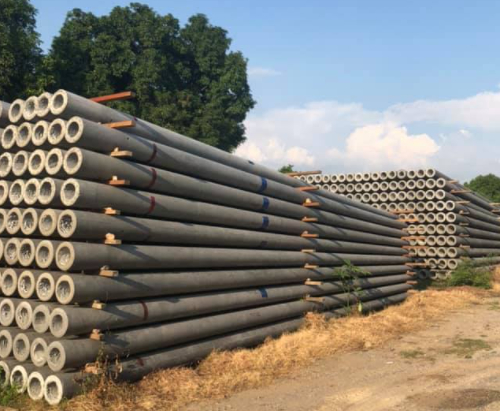Concrete Electric Pole, Length, Weight, Function, Concrete Pole Price List, RCC Pole, PCC Pole

# Concrete Electric Pole, Length, Weight, Function, Concrete Pole Price List, RCC Pole, PCC Pole

Summary

Concrete Electric Pole, Length, Weight, Function, Concrete Pole Price List, RCC Pole, PCC PoleConcrete Electric Pole, Length, Weight, Function, Concrete Pole Price List, RCC Pole, PCC Pole
In this article, I will discuss what is electric pole, working load of the electric pole, pre-stressed concrete, weight of electric pole, the diameter of the electric pole, depth of digging, etc. Each and everything I will try to explain in an easy way.
The electric poles are erected everywhere to transmit electricity.
But many people don't know the specifications of the electric pole.
The normally electrical pole which we use is PCC and RCC pole.
The full form of PCC pole is plain cement concrete and the full form of RCC pole is reinforced cement concrete. The plain cement concrete pole is made with cement and concrete, the PCC pole has low mechanical strength. The reinforced cement concrete is made with cement concrete and some rods to increase the strength of the pole, the RCC pole has high mechanical strength.
The electric poles are used to give support to the overhead line to transmit electricity from one point to another point.
The pole which is used nowadays for distribution is of 8 meters or 9-meter height.
8 Meter Electric Pole
The working load of the PCC electric pole is 200kg if the load increases beyond 200kg then the pole may get a break and the working load of the RCC pole is more 250 - 300 kg because rods are used to make this pole.
The weight of the PCC pole is 380 kg whereas the weight of RCC pole is more between 420 to 450 kg, the pole has three holes at the top to fix cross arm of diameter 18 mm as shown in the above figure. The depth of planting or digging for an 8-meter pole is 1.5m.
The top width of the electric pole is 145 mm and the bottom width is 290 mm as shown in the above figure.
9 Meter Electric Pole
The working load of the 9-meter PCC pole is 300 kg if the load goes beyond 300 kg then the pole may get damaged whereas the working load of the RCC pole is 350 to 400kg.
The weight of the 9-meter PCC pole is 470 kg and the weight of the RCC pole is somewhat more 600 kg.
The top width of the pole is 185mm and the bottom weight of the pole is 355 mm.
The reinforced cement concrete and plain cement concrete poles can be used up to 33 KV. Above 33 KV transmission towers are used.
The cost of an 8 meter RCC pole is 4,500 and for 9 meters the cost is 5,500 rupees.
The cost of an 8 meter PCC pole is 3,500 rupees and the cost of a 9 meter PCC pole is 4,500 rupees.
Tags: Concrete Electric Pole, Length, Weight, Function, Concrete Pole Price List, what is the cost of the electric pole, what is the height of the electric pole, what is the weight of the electric pole.